Search IntMath
Close

450+ Math Lessons written by Math Professors and Teachers

5 Million+ Students Helped Each Year

1200+ Articles Written by Math Educators and Enthusiasts

Simplifying and Teaching Math for Over 23 Years

# A Brief Overview of the Triangle Inequality

The triangle inequality states that the sum of the lengths of any two sides of a triangle must be greater than the length of the remaining side.

Consider triangle ABC below: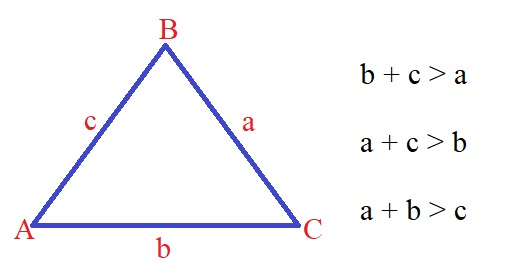The inequalities to the right are the three conditions of the triangle inequality that must be satisfied in order for side lengths a,b, and c to form a triangle. If one of the conditions fail, the side lengths cannot form a triangle.

# The Converse of the Triangle Inequality Theorem

It is not possible to construct a triangle from three line segments if any of them is longer than the sum of the other two.

In other words, no side of a triangle can be longer than the sum of the other two sides. This may seem like a simple concept, but it's actually a pretty important one! Let's take a closer look.

### The Triangle Inequality and You

If you've ever taken a geometry class, then you're probably already familiar with the triangle inequality. However, even if you haven't taken a formal geometry class, you've probably still encountered the triangle inequality in your everyday life.

For example, given any three side lengths, we can determine if the side lengths will form a triangle using the triangle inequality.

We can also use the triangle inequality to find the direct distance between two objects if given some other relevant conditions. We shall explore this more in the examples below.

## Practice Problems

Example 1

Which set of numbers could be the lengths of the sides of a triangle?

(A){12, 18, 30} (B) {12, 18, 24} (C) {6, 6, 14} (D) {2, 4, 6}

Solution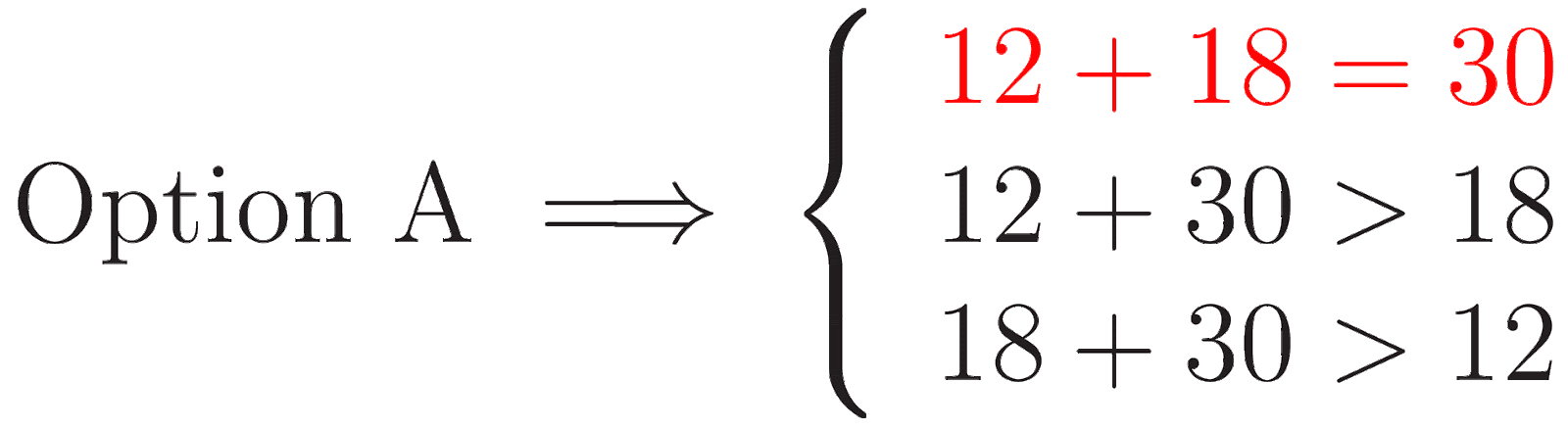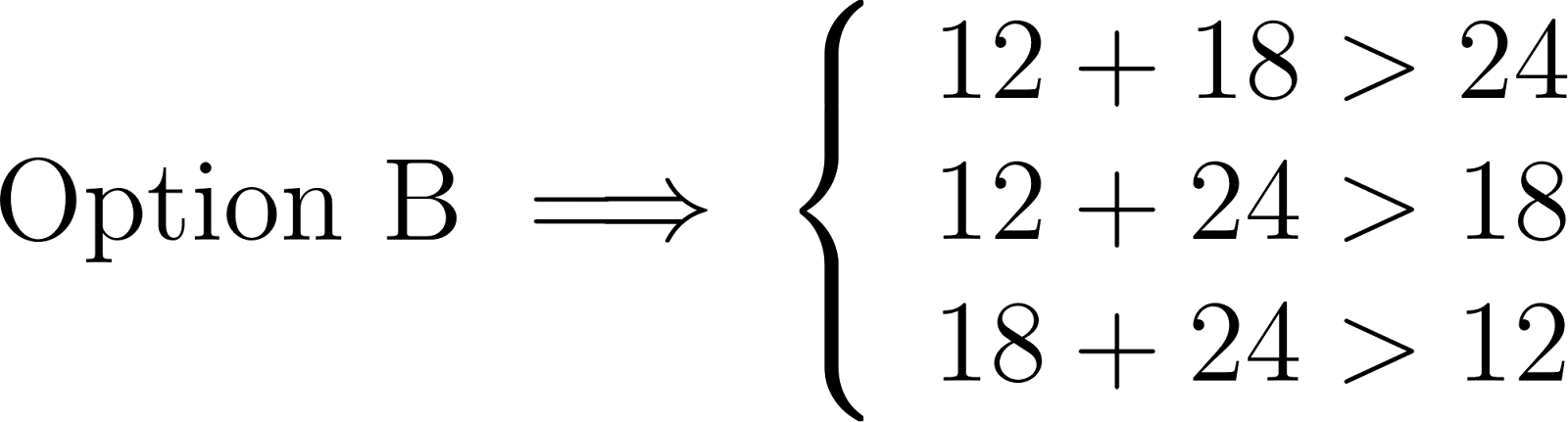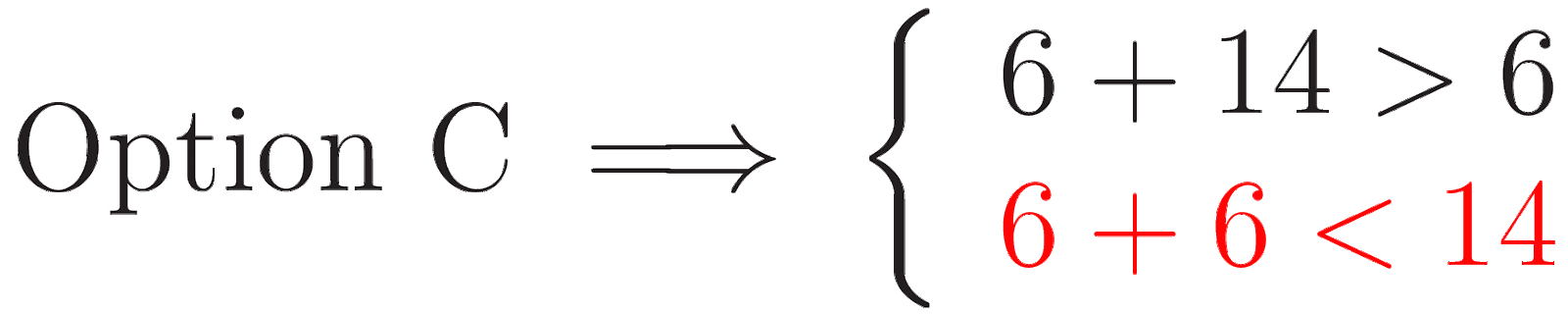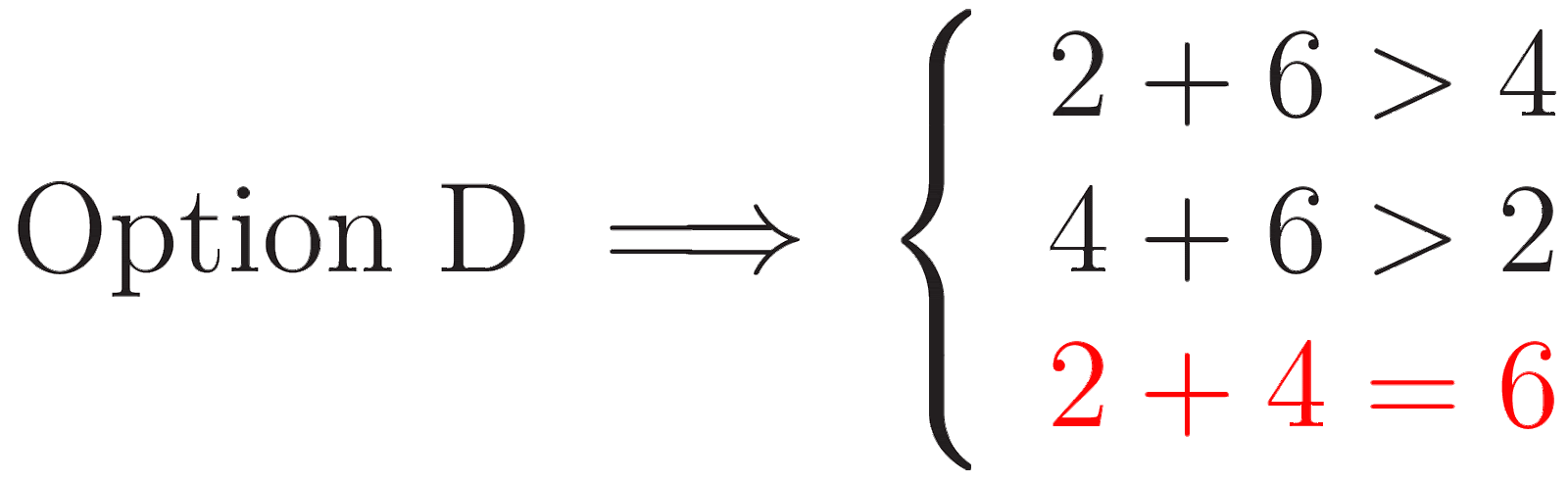The correct choice is B.

Example 2

Three boxes are placed on a table. The direct distance from Box A to Box B is 30 cm. The direct distance from Box B to Box C is 20 cm. Which of the choices could be the direct distance from Box A to Box C?

(A) 65 cm (B)55 cm  (C)35 cm (D) 5 cm

Solution

Let the direct distance from Box A to Box C = x

By the triangle inequality, we have the following inequalities: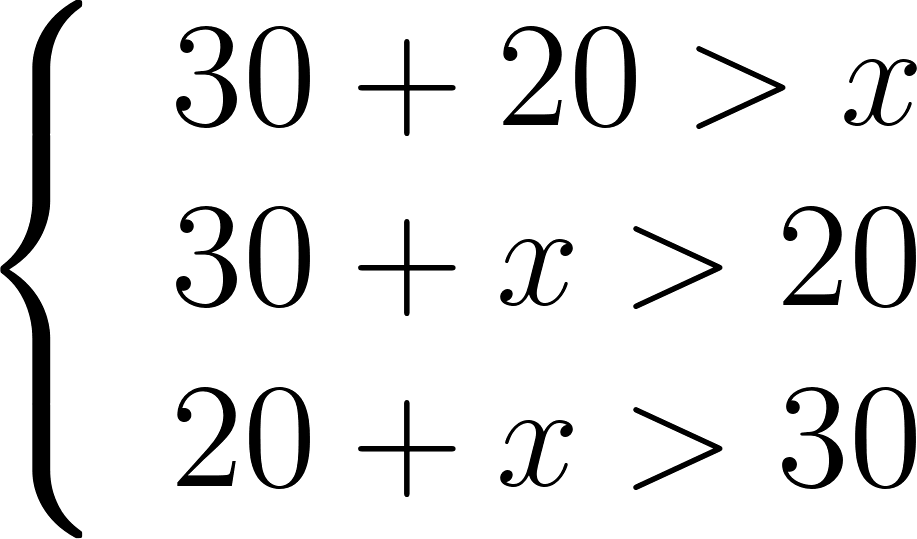Solve each of the inequalities for x: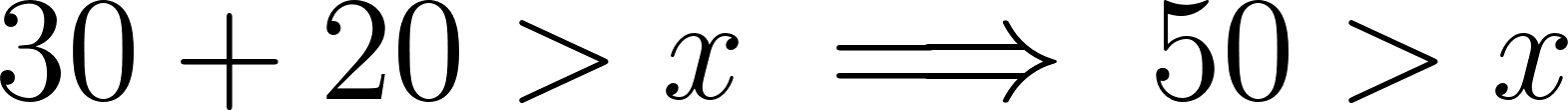Ignoring the negative value of x, we have that:Thus, of the given options, the possible direct distance from Box A to Box C is 35 cm. (Option C).

Example 3

In ΔPQR, PQ = 5 feet and QR = 3 feet. Which of the choices represents all possible values for PR, in feet?

(A)2<PR<8 (B)3<PR<7 (C)2≤PR<8 (D)3<PR≤7

Solution

By the triangle inequality, we have the following inequalities:Solve each of the inequalities for x:Ignoring the negative value of PR, we have that: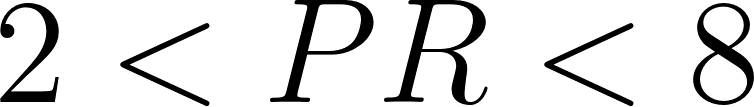Thus, the correct answer is Option A.

As you can see, understanding and being able to use the triangle inequality can come in handy in all sorts of everyday situations. Why not try the practice problems below?

## More Practice Problems

1. Find the possible lengths for the third side of a triangle with sides 20 and 18. Express your final answer as a compound inequality.
2. It is possible to construct a triangle with sides lengths 16, 33, and 13. True or False.
3. Which of the following set of numbers could not represent the three sides of a triangle (A) {13,20,34} (B) {8,20,30} (C) {10,18,23} (D) {14,23,36}
4. Which three lines of a given length can be three sides of a triangle? (A) 42mm, 22mm, 12mm (B)5cm, 50mm, 0.06m (C)10m, 5m, 50dm  (D)2.1cm, 4.2cm, 1.9cm
5. Two sides of an isosceles triangle measure 4 and 8. Which of the following choices could be the measure of the third side? (A) 10  (B)8  (C)6  (D)4

## FAQ

### How do you explain triangle inequalities?

There are a few different ways to approach explaining triangle inequalities, depending on what level of math understanding your audience has. If your audience is composed of students who are just learning about geometry, you might explain it in terms of the lengths of the sides of a triangle. If the audience understands more abstract concepts, you could approach it from the perspective of angles or sides.

### What is the triangle inequality theorem?

The triangle inequality theorem states that the sum of the lengths of the two shorter sides of a triangle is greater than the length of the longest side. This theorem can be used to write an inequality for a triangle.

### Why is the triangle inequality important?

The triangle inequality is a fundamental concept in geometry, and it has a number of applications in mathematics and physics. It is used to prove a number of other theorems, and it can be used to solve problems in a variety of fields.

### What are some applications of the triangle inequality?

The triangle inequality has a number of applications in mathematics and physics. It can be used to prove a number of other theorems, and it can be used to solve problems in a variety of fields. Some specific applications include finding the shortest path between two points, understanding the behavior of waveforms, and predicting the outcomes of elections.

### What are some common mistakes people make when using the triangle inequality?

Some common mistakes people make when using the triangle inequality include assuming that the theorem applies in all situations, not being aware of the limitations of the theorem, and not being aware of the potential for error.

### What is the importance of learning triangle inequality?

The triangle inequality is important for a number of reasons. First, it can help you understand other mathematical theorems. Second, it can help you avoid making mistakes when using other theorems. Finally, it can help you write inequalities for triangles, which can be helpful in a number of different situations. The triangle inequality is an important theorem that has a number of applications in everyday life. It is important to understand the theorem and how it applies to different situations. Additionally, it is important to be aware of the potential for error when using the triangle inequality. By understanding the theorem and its applications, you can avoid making mistakes and use the triangle inequality to your advantage.

### What is triangle inequality in graph theory?

The triangle inequality in graph theory states that the length of the shortest path between two vertices is less than or equal to the sum of the lengths of the shortest paths between those vertices and all other vertices in the graph.

## Problem SolverThis tool combines the power of mathematical computation engine that excels at solving mathematical formulas with the power of GPT large language models to parse and generate natural language. This creates math problem solver thats more accurate than ChatGPT, more flexible than a calculator, and faster answers than a human tutor. Learn More.